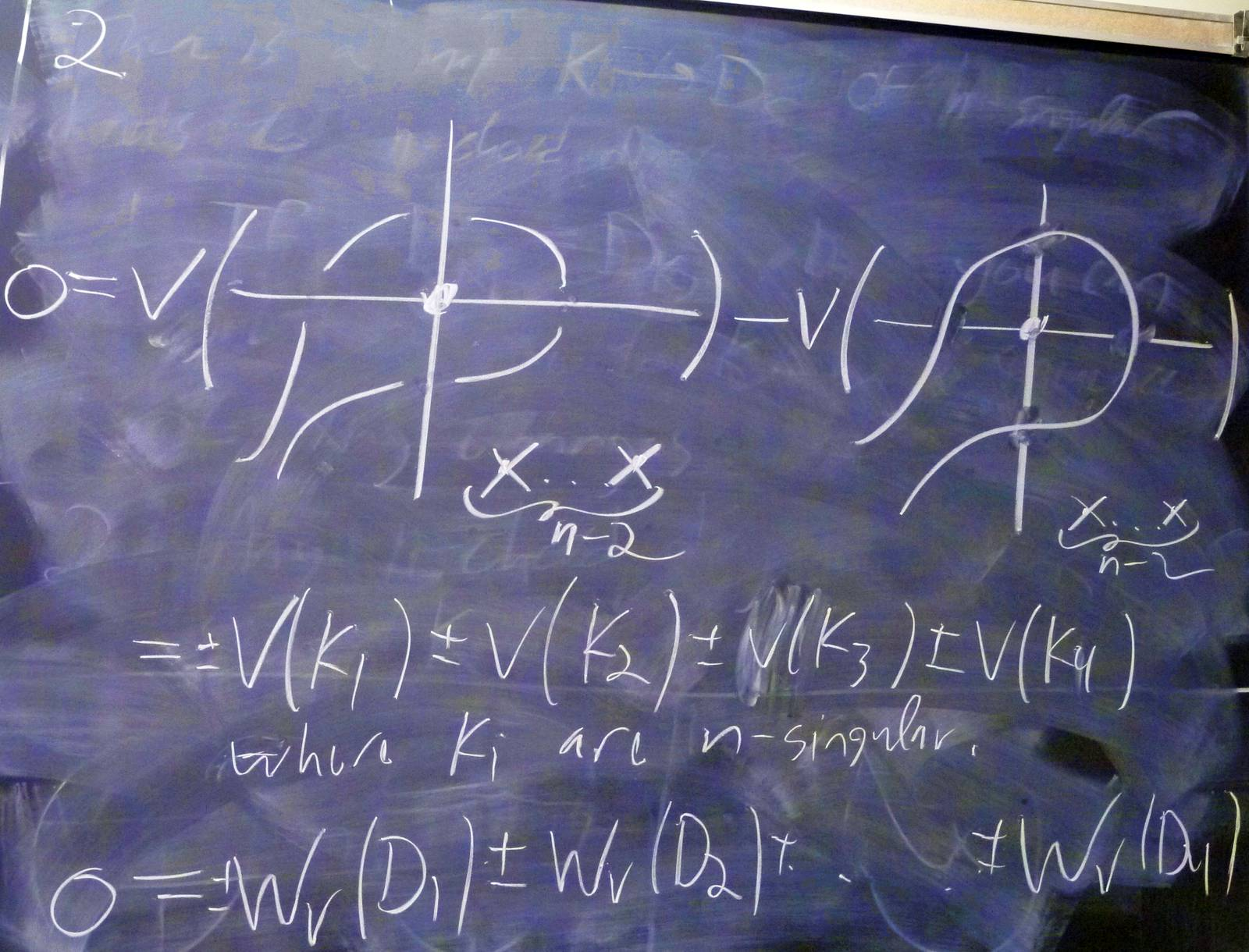© | Dror Bar-Natan: Classes: 2013-14: AKT: < >

# AKT-140127 Video

 width: 400 720 orig/AKT-140127.MOD For now, this video can only be viewed with web browsers that support

Notes on AKT-140127:    [edit, refresh]

Chord diagrams and weight systems.

# Week of... Notes and Links
1 Jan 6 About This Class (PDF).
: Course introduction, knots and Reidemeister moves, knot colourings.
Tricolourability without Diagrams
: The Gauss linking number combinatorially and as an integral.
: The Schroedinger equation and path integrals.
Friday Introduction (the quantum pendulum)
2 Jan 13 Homework Assignment 1.
: The Kauffman bracket and the Jones polynomial.
: Self-linking using swaddling.
: Euler-Lagrange problems, Gaussian integration, volumes of spheres.
3 Jan 20 Homework Assignment 2.
: The definition of finite-type and some examples.
: The self-linking number and framings.
: Integrating a polynomial times a Gaussian.
Class Photo.
4 Jan 27 Homework Assignment 3.
: Chord diagrams and weight systems.
: Swaddling maps and framings, general configuration space integrals.
: Some analysis of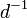$d^{-1}$.
5 Feb 3 Homework Assignment 4.
: 4T, the Fundamental Theorem and universal finite type invariants.
The Fulton-MacPherson Compactification (PDF).
: The Fulton-MacPherson Compactification, Part I.
: More on pushforwards,$d^{-1}$, and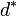$d^\ast$.
6 Feb 10 Homework Assignment 5.
: The bracket-rise theorem and the invariance principle.
: The Fulton-MacPherson Compactification, Part II.
: Gauge fixing, the beginning of Feynman diagrams.
R Feb 17 Reading Week.
7 Feb 24 : A review of Lie algebras.
: Graph cohomology and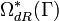$\Omega_{dR}^\ast(\Gamma)$.
: More on Feynman diagrams, beginning of gauge theory.
From Gaussian Integration to Feynman Diagrams (PDF).
8 Mar 3 Homework Assignment 6 (PDF)
: Lie algebraic weight systems.
: Graph cohomology and the construction of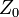$Z_0$.
Graph Cohomology and Configuration Space Integrals (PDF)
: Gauge invariance, Chern-Simons, holonomies.
Mar 9 is the last day to drop this class.
9 Mar 10 Homework Assignment 7 (PDF)
: The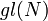$gl(N)$ weight system.
: The universal property, hidden faces.
: Insolubility of the quintic, naive expectations for CS perturbation theory.
10 Mar 17 Homework Assignment 8 (PDF)
: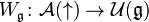$W_{\mathfrak g}\colon{\mathcal A}(\uparrow)\to{\mathcal U}({\mathfrak g})$ and PBW.
: The anomaly.
: Faddeev-Popov, part I.
Gaussian Integration, Determinants, Feynman Diagrams (PDF).
11 Mar 24 Homework Assignment 9 (PDF)
: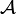${\mathcal A}$ is a bi-algebra.
: Understanding and fixing the anomaly.
Friday: class cancelled.
12 Mar 31 Monday, Wednesday: class cancelled.
: A Monday class: back to expansions.
E Apr 7 : A Friday class on what we mostly didn't have time to do.Add your name / see who's in!
Dror's Notebookrefresh
panel
Managed by dbnvp: You can click on many of the blackboard shots / frame grabs to see their full-size versions.

0:04:00 [add] Notes and reminders.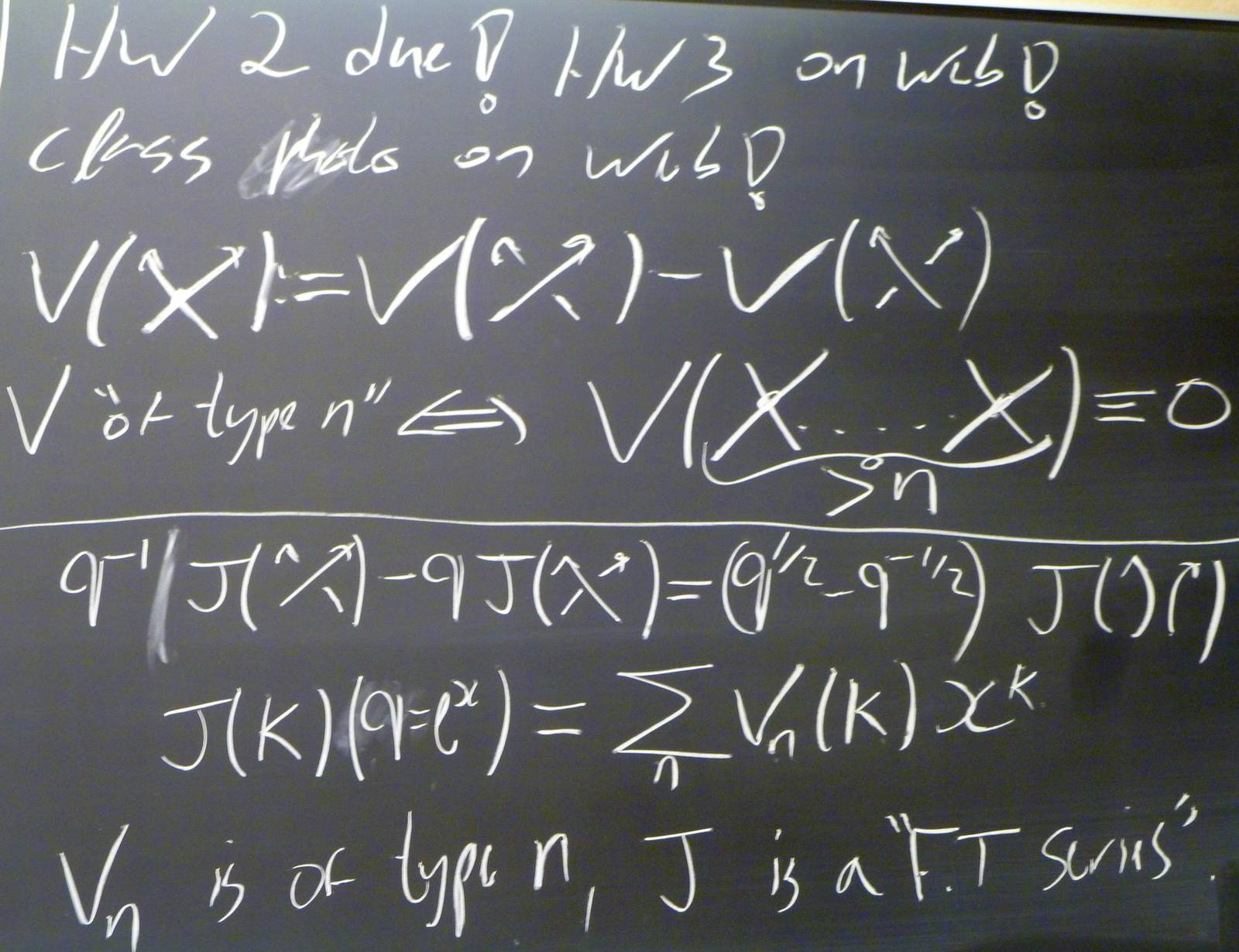0:13:21 [add] HOMFLY-PT and Conway.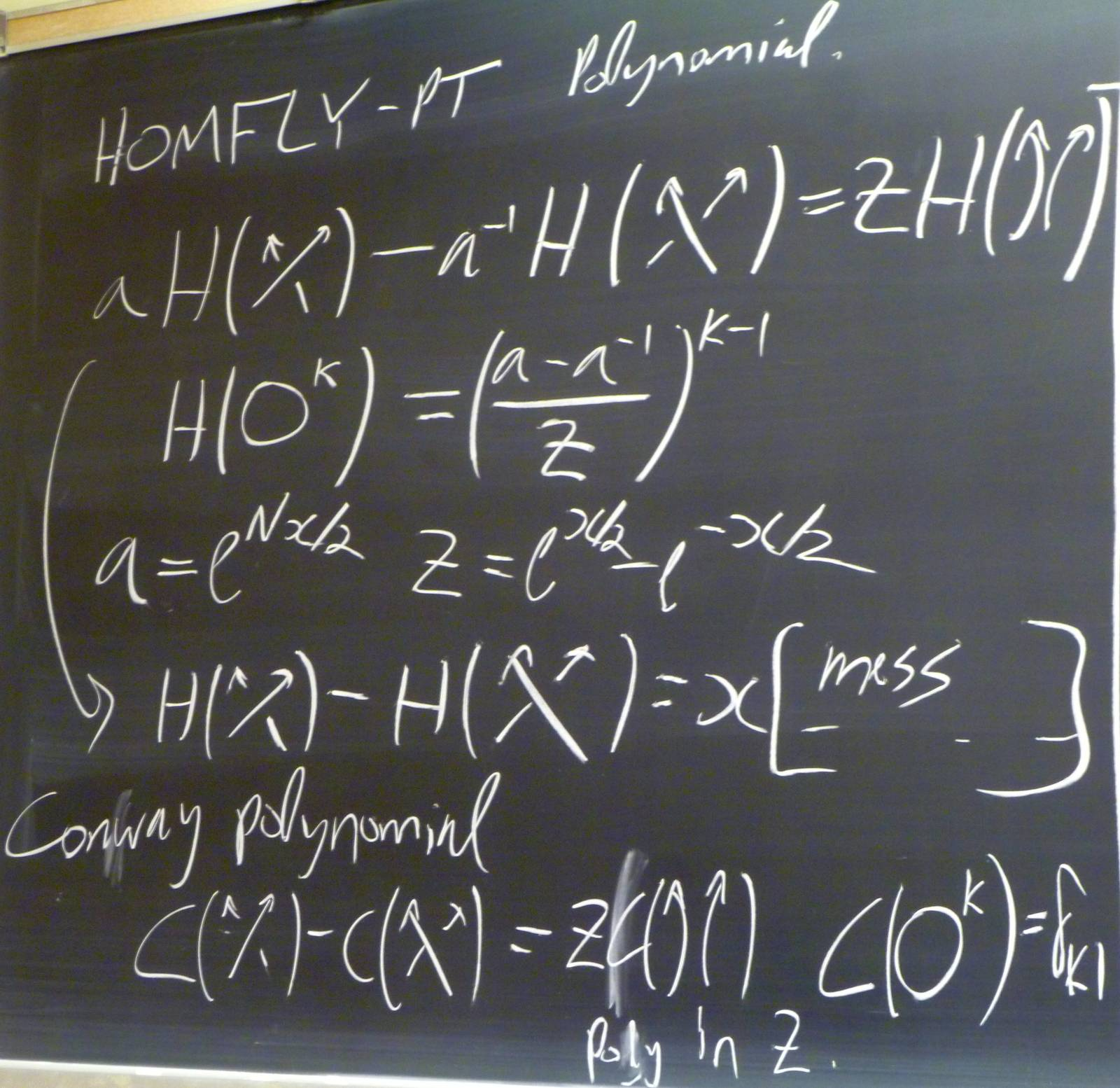0:23:46 [add] The $n$th derivative is constant.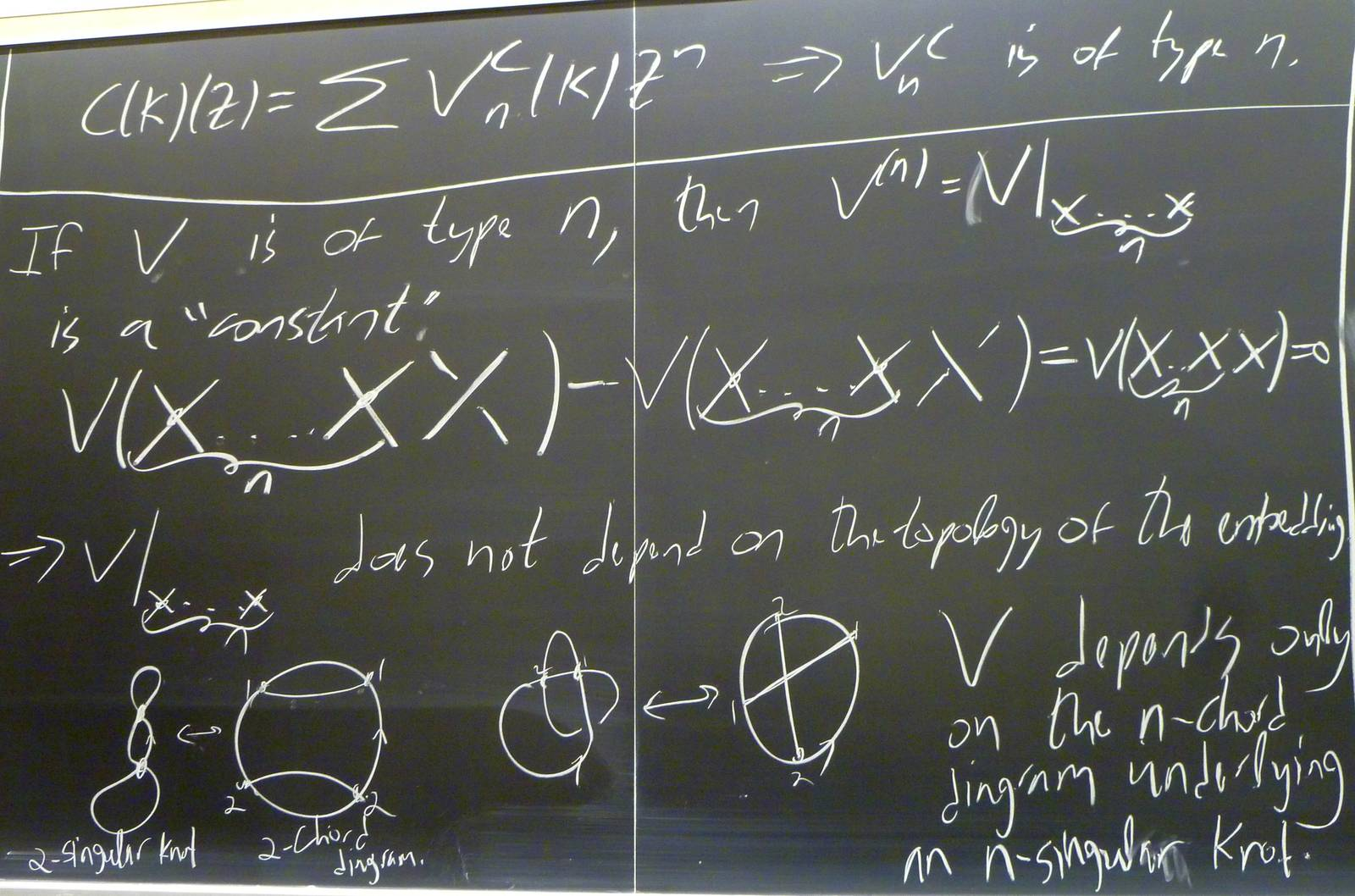0:25:24  Are chord diagrams unique? Or is the map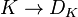$K \rightarrow D_{K}$ injective?

I think they are unique but not very sure.0:31:27  Much of this note comes from the paper "Finite type invariants of classical and virtual knots" by Goussarov, Polyak, and Viro, which can be found here: https://arxiv.org/abs/math/9810073.

Similar to the process of obtaining an n-chord diagram from an n-singular knot, there is a so called "Gauss diagram" obtainable from a regular knot. I.e. there is a map $\mathcal{K} \rightarrow \mathcal{D}$ from the space of knots into the space of Gauss diagrams. A Gauss diagram is a chord diagram with orientations and signs on the chords. The Gauss diagram of a specific knot is obtained as shown in examples below (the right-handed trefoil and the figure eight knots).

Note the additional information in the Gauss diagram about the overpasses and underpasses of the knot diagram (the orientations on the chords), as well as the sign of the crossings. Unfortunately, unlike the fact that any n-chord diagram is $D_K$ for some n-singular knot $K$, it is not true that any Gauss diagram is the Gauss diagram of a knot. This observation is intimately related to the theory of virtual knots, described in detail in the paper linked above.

0:32:16 [add] $n$-singular knots and $n$-chord diagrams.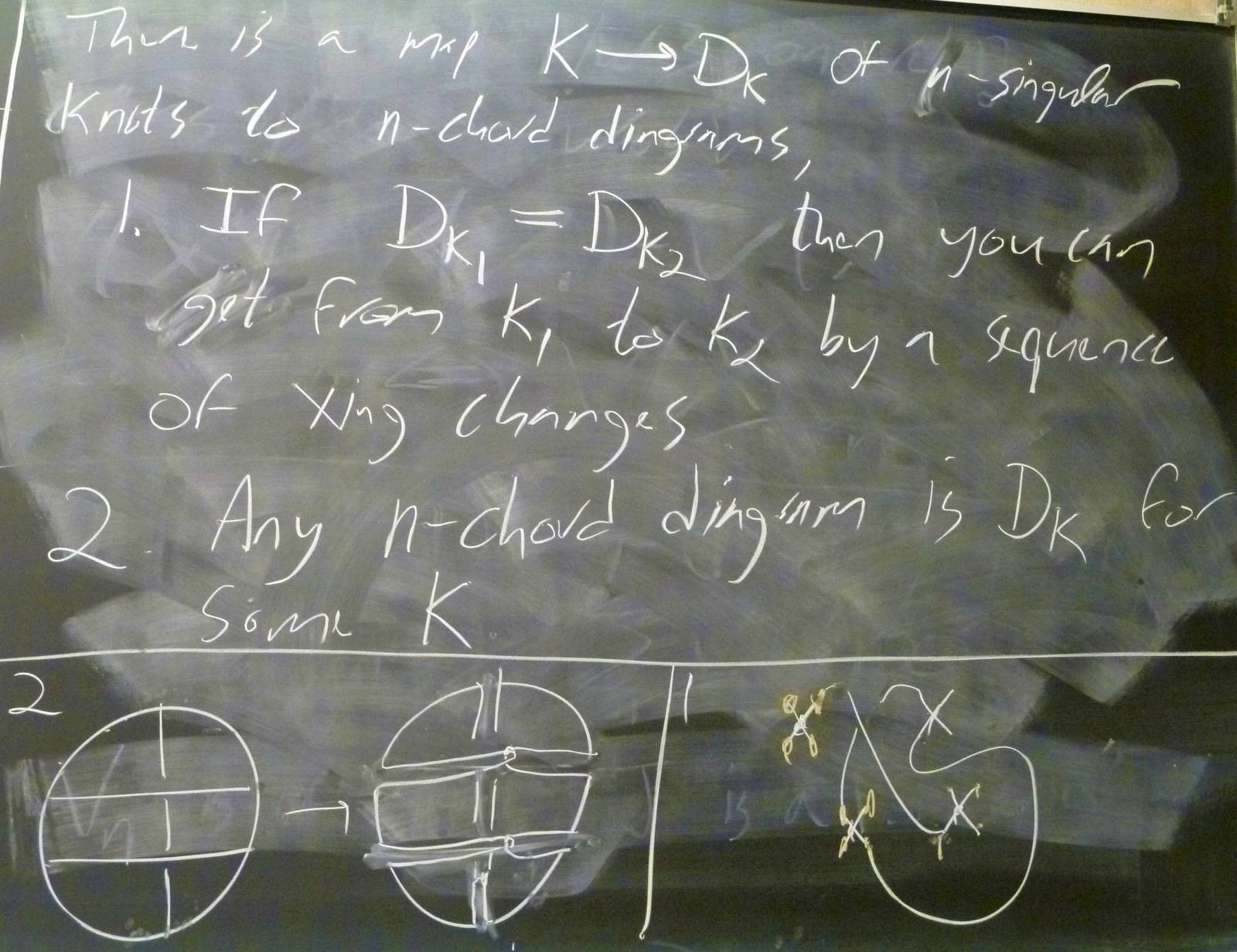0:44:35 [add] Weight systems and finiteness.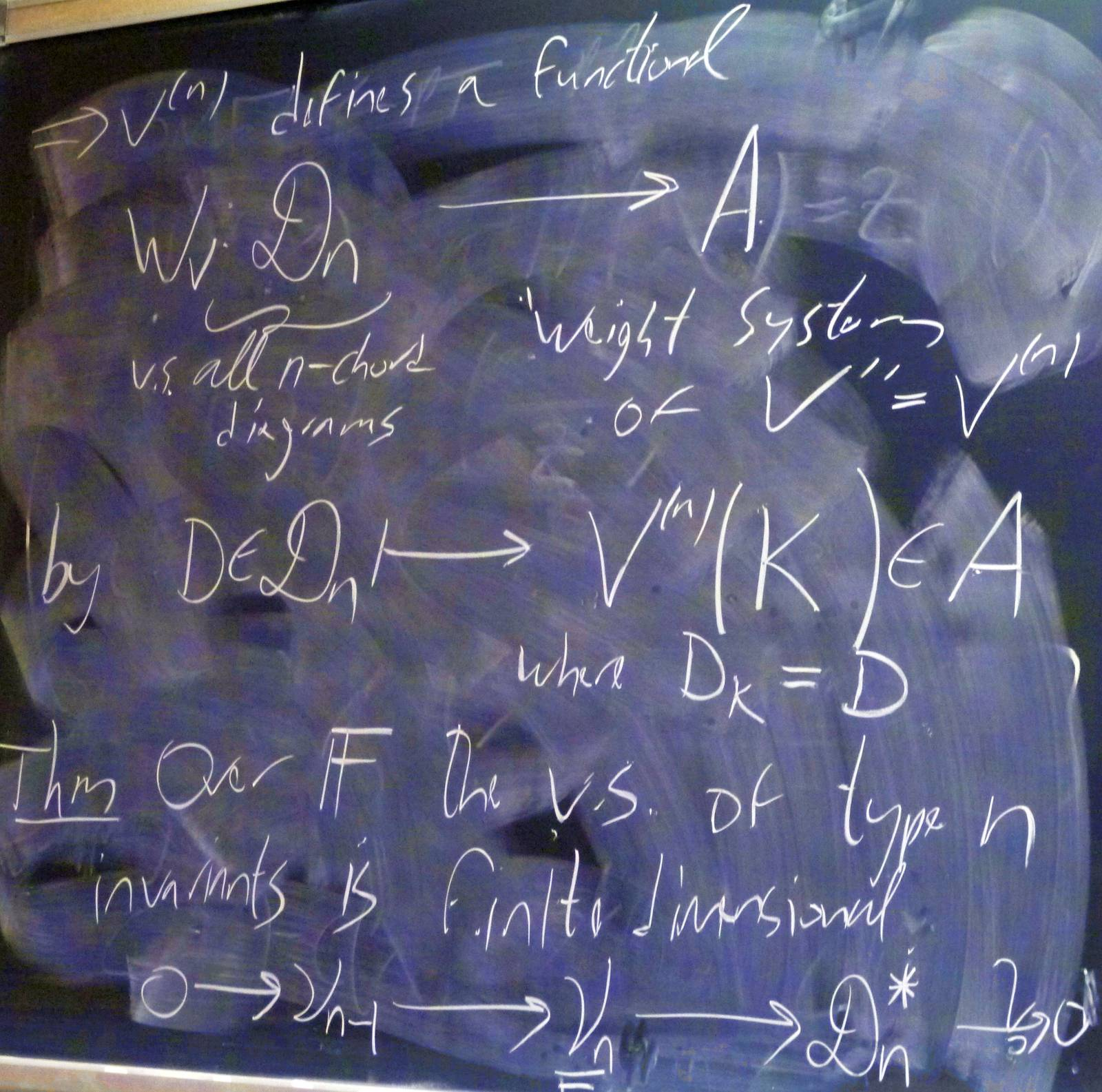0:47:32 [add] The FI relation.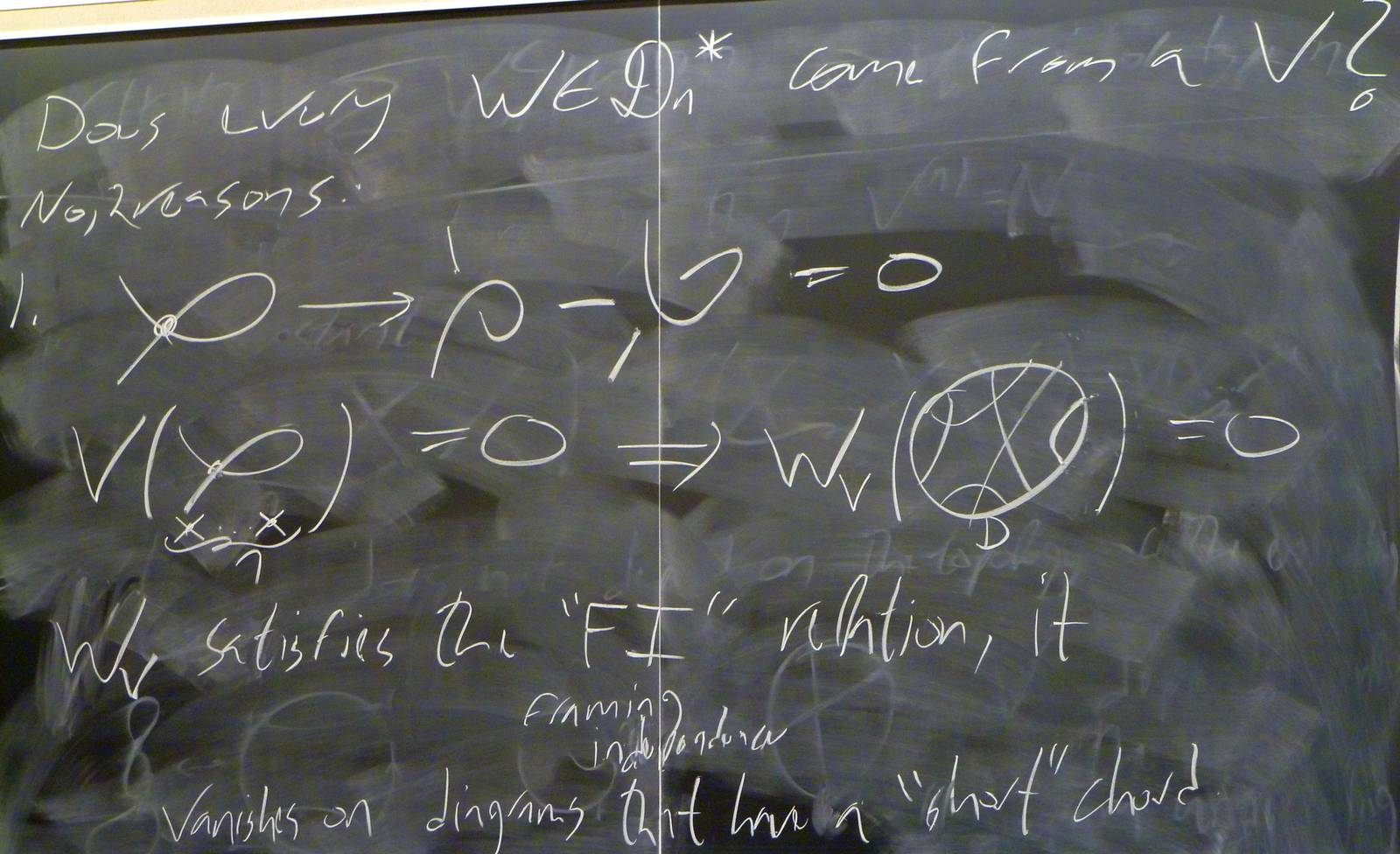0:47:34  This note closely follows the introductory parts of "Vassiliev and Quantum Invariants of Braids" by Dror Bar-Natan (1996).

The notion of finite type invariant can be extended to different knot-like objects (braids, tangles, links, etc.). While it is an open question whether finite type invariants separate knots, it is known that finite type invariants separate braids. In this note, we describe the process of getting a chord diagram from an m-singular braid, as well as describing the framework in which one would perform a similar analysis to the one done in lecture on braids.

Given a braid $B$ with n strands and m double points, the chord diagram $D_B$ is given as illustrated below (with $n$ = 4 and $m$ = 3).

It is clear how to do this for all $n$, $m$. The space of $m$-chord pure braid diagrams then forms a space $\mathcal{D}^{pb}_m$, and just as in the case of knots, given a type $m$ invariant $V$ of braids with $n$ strands with values in an abelian group $A$, there is a map $W_V: \mathcal{D}^{pb}_m \rightarrow A$ defined by $W_V(D) = V(B)$, where $D_B = D$. Then define $\mathcal{D}^{pb}$ as the completed direct sum of all $\mathcal{D}^{pb}_m$, and define $\mathcal{A}^{pb}$ as $\mathcal{D}^{pb}/\mathcal{I}$, where $\mathcal{I}$ is an ideal generated by relations among braid chord diagrams analogous to the FI and 4T relations of the familiar knot chord diagrams. If one continues with this analysis, one can find that finite type invariants in fact do separate braids. For a proof of this fact and much more detail regarding the above, see https://www.math.toronto.edu/drorbn/papers/glN/glN.pdf.

0:53:14 [add] The 4T relation.